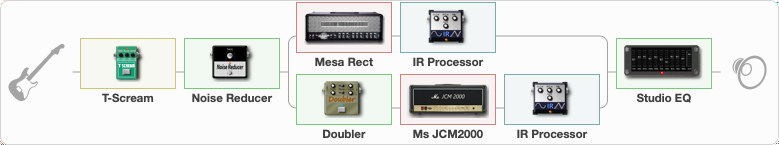# ToLiveIsToDie

Discussion in 'ToneLib-GFX presets' started by Serpov, Aug 1, 2022.

1. ToLiveIsToDie

Preset name: ToLiveIsToDie

Effects chain:Effect: "T-Scream" (Overdrive / Distortion), active - "yes"
{
"Drive" = 22
"Tone" = 80
"Level" = 79
}

Effect: "Noise Reducer" (Dynamics / Filter), active - "yes"
{
"Sens" = 73
"Mode" = Soft
}

Effect: "Splitter" (Dynamics / Filter)
{
"A-Bypass" = Off
"A-Pan" = -100
"A-Level" = 55
"B-Bypass" = Off
"B-Pan" = 100
"B-Level" = 55
"Width" = 0

'A' branch:
{

Effect: "Mesa Rect" (Amp simulators), active - "yes"
{
"Gain" = 50
"Bass" = 60
"Middle" = 32
"Treble" = 86
"Presence" = 78
"Master" = 62
"Level (dB)" = 0
}

Effect: "IR Processor" (Cabinets), active - "yes"
{
"IR" = tolive2-001
"Low Cut (Hz)" = 0
"Hi Cut (kHz)" = 20.0
"Mix" = 100
"Level (dB)" = 0
}
}
'B' branch:
{

Effect: "Doubler" (Dynamics / Filter), active - "yes"
{
"A-Dly" = 72
"A-Inv" = Off
"A-Level" = 100
"B-Dly" = 22
"B-Inv" = On
"B-Level" = 100
}

Effect: "Ms JCM2000" (Amp simulators), active - "yes"
{
"Gain" = 50
"Bass" = 65
"Middle" = 38
"Treble" = 77
"Presence" = 83
"Master" = 52
"Level (dB)" = 0
}

Effect: "IR Processor" (Cabinets), active - "yes"
{
"IR" = justice_R_IR
"Low Cut (Hz)" = 0
"Hi Cut (kHz)" = 20.0
"Mix" = 100
"Level (dB)" = 0
}
}
}

Effect: "Studio EQ" (Dynamics / Filter), active - "yes"
{
"31 Hz" = 0
"62 Hz" = 0
"125 Hz" = 6
"250 Hz" = -2
"500 Hz" = -3
"1 kHz" = 6
"2 kHz" = 4
"4 kHz" = 1
"8 kHz" = 0
"16 kHz" = 0
"Level (dB)" = 0
}

Note: You will need to download and install the ToneLib-GFX software to use the preset.

#### Attached Files:

• ###### ToLiveIsToDie.tlgfx
File size:
8.4 KB
Views:
1,847
karlosnoir, badtz_maru, qred and 3 others like this.